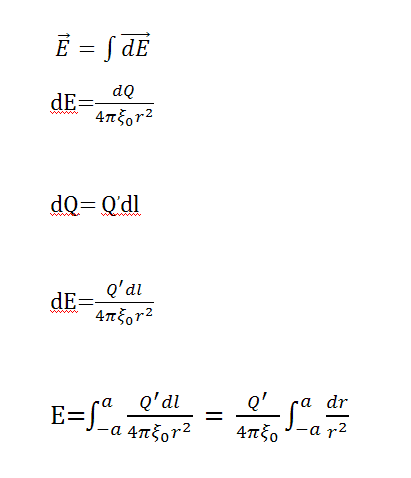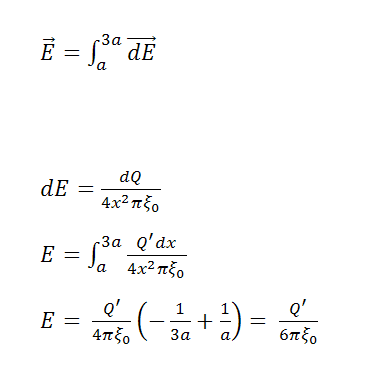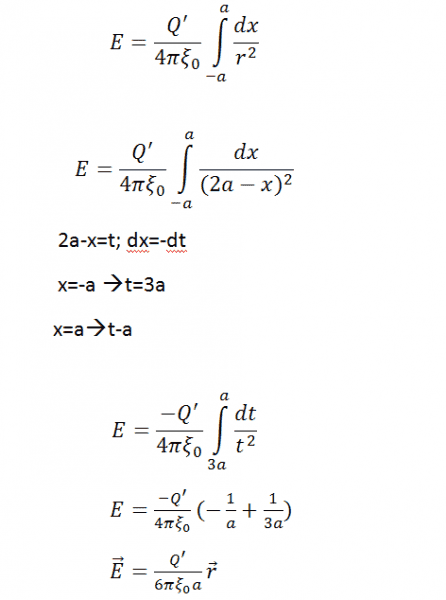# Electric field at specific point

< Mentor Note -- thread moved to HH from the technical engineering forums, so no HH Template is shown >

Determine the electric field in point P with coordinates (2a,0), if there's thin rod charged with electric charge Q and it's laying on x coordinate with lenght from -a to a, and it it's placed in vacuum.

Last edited by a moderator:

If it is a thin rod then you may assume a linear charge distribution in the segment [-a,a]. I assume this distribution is uniform. Try to express an infinitesimal portion of the Electric field created at point P (## d\vec E## ) by an infinitesimal charge (## dq ##) at arbitrary position x in [-a,a]. I'll leave you to think a bit more about it.

If it is a thin rod then you may assume a linear charge distribution in the segment [-a,a]. I assume this distribution is uniform. Try to express an infinitesimal portion of the Electric field created at point P (## d\vec E## ) by an infinitesimal charge (## dq ##) at arbitrary position x in [-a,a]. I'll leave you to think a bit more about it.

I think i know what you mean, i'll try to solve it by summing (integrating) contributions of all dE vectors, when i solve it i'll post it here.

•Demforiq
If it is a thin rod then you may assume a linear charge distribution in the segment [-a,a]. I assume this distribution is uniform. Try to express an infinitesimal portion of the Electric field created at point P (## d\vec E## ) by an infinitesimal charge (## dq ##) at arbitrary position x in [-a,a]. I'll leave you to think a bit more about it.

I tried integrating it but every time i end up with this:But i know it's not correct since result is zero so i probably made a mistake but i can't find where.

That is what I meant. Still, there are some issues in your work:
1. Your second equation should have vectors to be coherent with what you are doing in the first one (just multiply by the unitary vector that has the direction of the line that goes from the charge to the point P). Luckily, all the contributions to the electric field are parallel, so the vector will be constant in the integral.
2. Why use ##dl## when your rod is parallel to coordinate ##x##?
3. Be aware that ##r## is the distance from the charge (##dQ##) to point ##P##. Can you express it as a function of ##x##?
But i know it's not correct since result is zero so i probably made a mistake but i can't find where.
You should not arrive to this integral if you do it right, but I must tell you that it is not zero: actually it is a divergent improper integral (note that ## r \rightarrow 0 \Rightarrow \frac{1}{r^2} \rightarrow \infty ## ).

Check http://en.wikipedia.org/wiki/Improper_integral

That is what I meant. Still, there are some issues in your work:
1. Your second equation should have vectors to be coherent with what you are doing in the first one (just multiply by the unitary vector that has the direction of the line that goes from the charge to the point P). Luckily, all the contributions to the electric field are parallel, so the vector will be constant in the integral.
2. Why use ##dl## when your rod is parallel to coordinate ##x##?
3. Be aware that ##r## is the distance from the charge (##dQ##) to point ##P##. Can you express it as a function of ##x##?

You should not arrive to this integral if you do it right, but I must tell you that it is not zero: actually it is a divergent improper integral (note that ## r \rightarrow 0 \Rightarrow \frac{1}{r^2} \rightarrow \infty ## ).

Check http://en.wikipedia.org/wiki/Improper_integral

So, basically i can use dx instead of dl since rod is parallel to the x coordinate?

Exactly: a piece of ##l## is a piece of ##x##.

So, is this better:Yes but your integration limits are incorrect. You should be integrating (summing) contributions to the electric field of charges within the rod, not outside (##[a,3a]## is outside).
Furthermore, you should have the distance from the charge ##dQ## to the point P (that is, ##r##) expressed as a function of ## x ## (note that it is not identical to ##x##). Just to make things clearer, include the unitary vector:

## \vec {dE} = \frac {Q' dx}{4 r^2 \pi \epsilon_0} \vec i ##

where ## \vec i ## is the unitary vector in the ##x ## direction (remember that the electric field is a vector).

Last edited:
Yes but your integration limits are incorrect. You should be integrating (summing) contributions to the electric field of charges within the rod, not outside (##[a,3a]## is outside).
Furthermore, you should have the distance from the charge ##dQ## to the point P (that is, ##r##) expressed as a function of ## x ##

## \vec {dE} = \frac {Q' dx}{4 r^2 \pi \epsilon_0} \vec i ##

where ## \vec i ## is the unitary vector in the ##x ## direction (remember that the electric field is a vector).

I know it's a vector but it makes no difference while calculating it, i see, i should put integration limits [-a,a] since that is the part with the electric field. I'll finish it and post it. Thank you.

I know it's a vector but it makes no difference while calculating it
Correct, since all contributions are parallel. Anyway, it does no harm. ; )

Correct, since all contributions are parallel. Anyway, it does no harm. ; )

It would be different if point P coordinates were for example (2a, 2a), then there would be two components of a vector dE, anyway i tried to express r as a function of x and i got r=2a-x and this is what i got:Where r' represents unitary vector (i hope 'unitary' is the correct term) that defines direction of the vector E'. Hopefully, this is correct, if it isn't please point it out, anyway i think i understand this kind of problems better now, thank you :)

Seems ok to me. Just to complete the problem, try to express ## Q' ## in terms of given data (##a ## and ##Q##).
Where r' represents unitary vector (i hope 'unitary' is the correct term) that defines direction of the vector E'.
I think 'unitary' is a bad translation... It is actually 'unit' vector: I'm sorry.
thank you:)